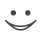# Get these flashcards, study & pass exams. For free! Even on iPhone/Android!

## Semester Exam Part 3 of 3 (24 Cards)

7. What does the motion of an object look like after the forces acting on it end? (Lab 15.6)
It stops moving.
8. What are the two conclusions that make up Newton’s First Law of Motion?
•  an object in motion stays in motion unless acted
Upon by an exterior force
•vice versa
9. What would constitute a violation of Newton’s First Law of Motion?
The air puck lab.
10. Explain Newton’s Second Law of Motion in your own words and provide several examples of the Law.
second law of motion: the rate of change of momentum is proportional to the imposed force and goes in the direction of the force.

Examples are found in everyday life, such as with forces acting upon a box- it moves in the direction that is the mean of the signed numbers.
11. A Lexus automobile has a mass of 1,748 kg.  Starting from rest, it is able to reach a velocity of 26.8 m/s in 7.5 seconds.  What is the average force applied to the Lexus as it goes from 0 to 26.8 m/s?
83,698.9013 J/s
12. A Corvette has a mass of 1, 645 kg.  If it starts from rest, it can reach 26.8 m/s in 7.8 seconds.  What is the average force applied to the Corvette as it goes from 0 to 26.8 m/s?
80,479.7128 J/s
11. A Lexus automobile has a mass of 1,748 kg.  Starting from rest, it is able to reach a velocity of 26.8 m/s in 7.5 seconds.  What is the average force applied to the Lexus as it goes from 0 to 26.8 m/s?
11. A Lexus automobile has a mass of 1,748 kg.  Starting from rest, it is able to reach a velocity of 26.8 m/s in 7.5 seconds.  What is the average force applied to the Lexus as it goes from 0 to 26.8 m/s?
1. Differentiate between balanced and unbalanced forces.
A balanced force Is a force whose sum of vectors equals 0; an unbalanced force is one whose sum of vectors is not equal to zero.
2. Define a vector and give examples.
A vector shows the direction and magnitude of a force. The equation is l=k•force, such as 12cm or 22112 cm.
3. What is the connection between the length/direction of a vector and the force?
L=k•force
4. Using a proportionality constant of 0.2 cm/N, complete the following chart.
 Size of Force (N) Length of vector (cm) 5 1 16 3.2 3.5 0.7 28 5.7
What is net force and how is it calculated?
Net force is the combination of signed numbers; 'tis calculated by adding the signed numbers.
6. What do we call the two forces that add to form a single force?
Components
1. Read a distillation graph to determine the properties of the fractions from the mixture. (Exp. 5.1).
Okay, I did it!
2. On a distillation graph, how do you distinguish between the different substances?
You determine them by their boiling point.
3. Explain the method Separation by flotation.
Froth flotation is a process for selectively separating hydrophobic materials from hydrophilic. This is used in several processing industries. Historically this was first used in the mining industry.
4. What does the term filtrate refer to?
Passing a combination of minerals through a filter to filtrate  only what you want.
5. What must be different in order to separate solids using the filtration method?
The size of the filter.
6. Explain the process of fractional crystallization.
Fractional crystallization (chemistry), a process to separate different solutes from a solution
7. What technique can be used when separating a mixture that contains only a minute amount of a substance?
Decanting
8. How would you eliminate the carbon dioxide that may be dissolved in a sample of water?
Fractional distillation.
9. Compare and contrast mixtures and pure substances and ways to identify them.
Mixtures contain 2 or more pure substances; pure substances keep their characteristics in any condition*.
THE END
You can go home now.Flashcard set info:
Author: CoboCards-User
Main topic: Science
Topic: Physics
City: P
Published: 05.06.2012

Card tags:
All cards (24)
no tagsReport abuse